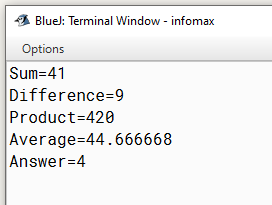# Java program using int variables to find the sum of three numbers say 15 36 and 45 and subtract the result from 100 using variables

Write a program using int variables to find the sum of three numbers say 15, 36 and 45 and subtract the result from 100 using variables.

`Solution`

``````class Demo
{
public static void main(String arr[])
{
int a=15,b=36,c=45,d;
d=100-(a+b+c);
`Output`